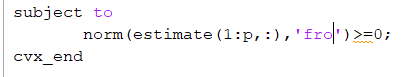# Cvx is giving error when i use this inequality constraint,why?norm(x)>=constant is not a convex constraint. The fact that your constraint is trivial because constant=0 is a coincidence.

1 Like

i thought all norms are convex

`convex_expression <= constraint` is a convex constraint. `convex_expression >= 0` is non-convex.

Also, norm(affine_expression) is convex, but norm(non_affine_expression) is not necessarily.